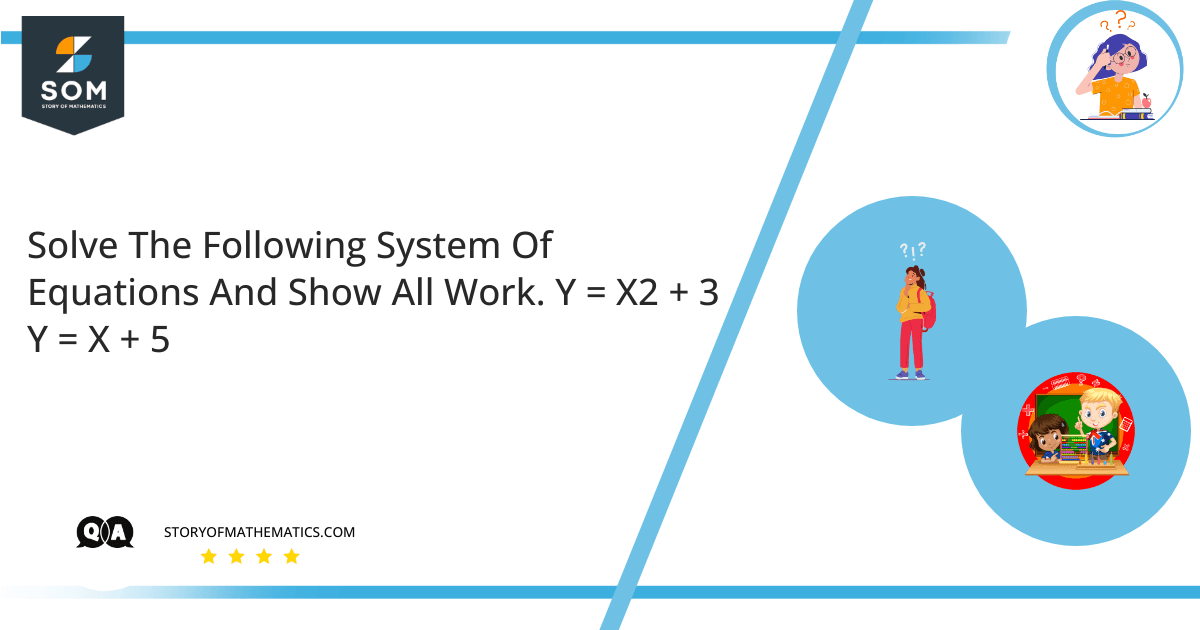# Solve the system of equations and show all work.1. y = x^2 + 3
2. y = x + 5
• This question aims to solve the linear equation system and calculate the variable’s values. In mathematics, a set of simultaneous equations, also known as a system of equations or equation systems, is a limited set of mathematics equations required by the exact solutions. The mathematical system is usually divided in the same way as single statistics, namely:
•
• System of nonlinear equations
• System of linear equations
• System of the bilinear equation
• System of differential equations
• System of difference equation

A system of linear equations is a defined combination of one or more linear equations having the same variable. In mathematics, line programming theory is a fundamental component of linear algebra, a term used in many parts of modern mathematics. Computer algorithms for finding solutions are an integral part of algebra in the number line and play an important role in engineering, physics, chemistry, computer science, and economics. A non-line mathematical system can usually be measured by a line system, a helpful method for modeling a mathematical model or comparing a computer system with a relatively complex one.

Generally, mathematical coefficients are real or complex numbers, and solutions are searched in a set of the same numbers. Still, the theory and algorithms apply to coefficients and solutions in any field. Some ideas have been made to find answers in an important domain, such as the ring of whole numbers or other algebraic structures; see the line number above the ring. Integer linear programming is a set of methods for finding the “best” number solution (if there are many). Gröbner’s core theory provides algorithms in which coefficients and anonymity are polynomials. And the geometry of the tropics is an example of line algebra in an unusual structure.

The line system solution is the numerical value of the variables $x_[{1}, x_{2}, …, x_{n}$ to satisfy each figure. The set of all possible solutions determines the solution set of the equations.

The line system can work in any of three possible ways:

The system has complete solutions.

-The program has one unique solution.

-The system has no solution.

Solving these two equations give us:

$y=x^{2}+3$

$y=x+5$

$x^{2}+3=x+5$

$x^{2}-x=5-3$

$x^{2}-x=2$

$x^{2}-x-2=0$

$x^{2}-2x-x-2=0$

$x(x-2)+1(x-2)=0$

$(x+1)(x-2)=0$

$x+1=0 \:or\: x-2=0$

$x=-1\: or \: x=2$

$x=-1,2$

## Numerical Results

Solving the system of two equations gives values of $x=-1,2$.

## Example

Solve the system of equations as shown below and show all work.

$x+y=8$

$2x+y=13$

Solution

Solving these two equations gives us:

$x+y=8$

$2x+y=13$

$y=8-x$

$y=13-2x$

$x^{2}+8=x-3$

$8-x=13-2x$

$-2x+x=8-13$

$-x=-5$

$x=5$

$y=8-x$

$y=8-5$

$y=3$

$x=5\: or \:y=3$

$x=5 \:and\: y=3$

Solving the system of two equations gives the value of $x=5 \:and \:y=3$.